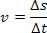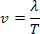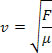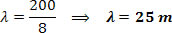Sound and Oscillation

# Velocity of propagation of a wave

In the sequence we see that while C travels a wavelength, the point P makes an oscillation

In physics, we say that the wave originates in elastic media such as strings, the surface of water, etc. Therefore, we define a wave as an oscillatory movement that propagates in a medium; being that in these movements only energy is transferred, that is, there is no transport of matter.

We know that there are two types of waves and they are classified as mechanical waves and electromagnetic waves. We distinguish a mechanical wave from an electromagnetic wave basically as follows:

– mechanical wave results from deformations caused in material means, that is, a mechanical wave needs a material medium to propagate .
– Electromagnetic wave is the result of the vibration of electric charges, that is, electromagnetic wave does not need a material medium to propagate. Therefore, an electromagnetic wave propagates in a vacuum.

For the propagation of a wave, we can use the same concept for calculating the average speed:We can deduce the speed with which a wave propagates; for that, we just have to do the quotient between the space in which the wave travels as a function of time. Let’s analyze the figure above, where we have the propagation of a wave. In it we can see that while point C travels a wavelength, each point of the string performs a complete oscillation.

For this reason, we can say that the time taken to travel a wavelength is the period T of the wave itself. Thus, based on the expression above, while point C travels a distance Δs = λ , the time taken is Δt = T . Thus, the propagation speed of a wave is given by:Or we can write it like this, as T = 1/f, we have:

v=λ .f

If the source producing the wave is simple harmonic, the period and frequency will be constant. Thus, we can say that the propagation speed of a wave on a string is given by:In the above equation we have:

– F is the tension in the string
– μ is the linear density of the string

Let’s see the following example : Suppose a wave has a frequency of 8 Hz and is propagating with a speed equal to 200 m/s. Determine the wavelength of the wave.

Removing the data provided by the exercise, we have: f = 8 Hz and v = 200 m/s, applying the fundamental equation of waves, we have:

v=λ .f

200=λ.8